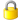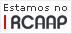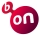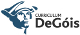Please use this identifier to cite or link to this item: `http://hdl.handle.net/10174/16983`

 Title: On the spectra of certain matrices and the iteration of quadratic maps Authors: Bandeira, LuísRamos, Carlos Correia Editors: Amat, Sergio Keywords: SpectrumQuadratic mapsIteration Issue Date: Jan-2015 Publisher: SeMA Journal Citation: On the spectra of certain matrices and the iteration of quadratic maps, L. Bandeira, C. Correia Ramos, SeMA J. 67 (2015), 51-69 Abstract: We study a sequence of one-parameter matrices, A_k(c), with k∈N and parameter c∈C, which is obtained recursively. For the given recursion, the characteristic polynomial of A_k(c) is given by −f^k_c(x), where f_c(x)=c−x^2 and f^k_c(x)=f_c∘⋯∘f_c(x). Therefore, the spectrum of each matrix A_k(c) corresponds to the pre-images of 0 under iteration of f_c. We obtain the block structure and a recursion for the eigenvectors of A_k(c), k=0,1,…. The structure of the eigenspaces of A_k(c) change dramatically with the parameter c, in particular their dimension as real linear spaces. For c∈R, we extensively use symbolic dynamics for unimodal maps of an interval to study this problem. We show that the logarithm of the growth rate of the real eigenspaces dimensions is equal to the topological entropy of f_c. Moreover, for c∈C, the relation between the sequence A_k(c) and the iteration of fc gives us an interesting interpretation of the spectrum of A_k(c) as the Julia set of f_c. URI: http://link.springer.com/article/10.1007/s40324-014-0026-5#http://hdl.handle.net/10174/16983 Type: article Appears in Collections: CIMA - Publicações - Artigos em Revistas Internacionais Com Arbitragem Científica

Files in This Item:

File Description SizeFormat
spectra_iteration_preprint_SeMA_rev4.pdf338.02 kBAdobe PDFView/OpenDSpace Software, version 1.6.2 Copyright © 2002-2008 MIT and Hewlett-Packard - Feedback